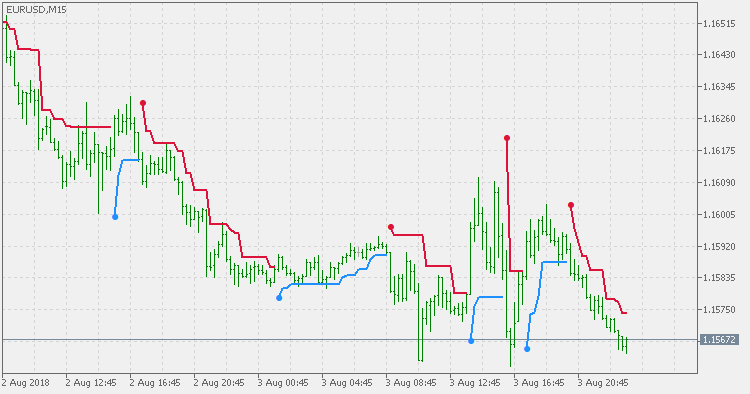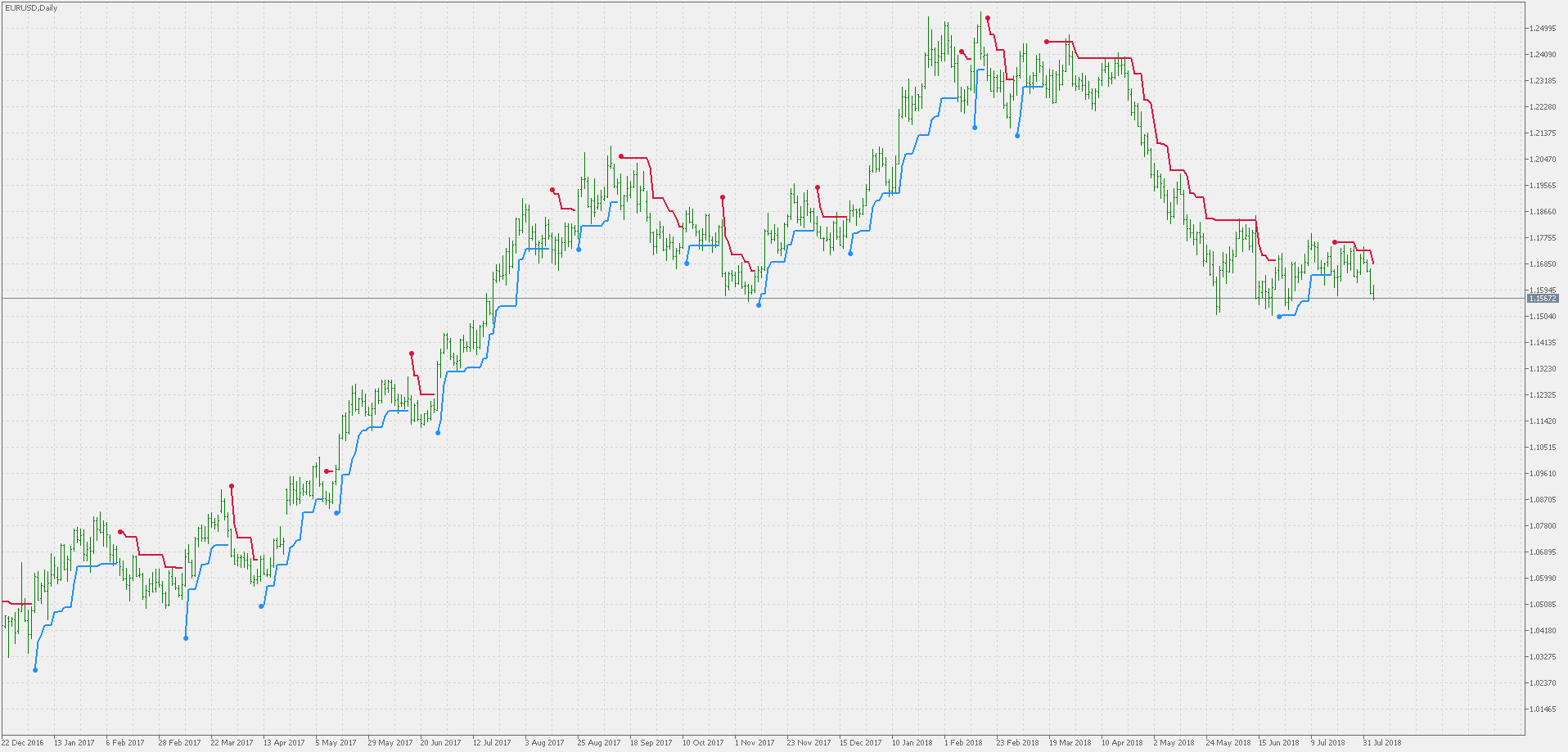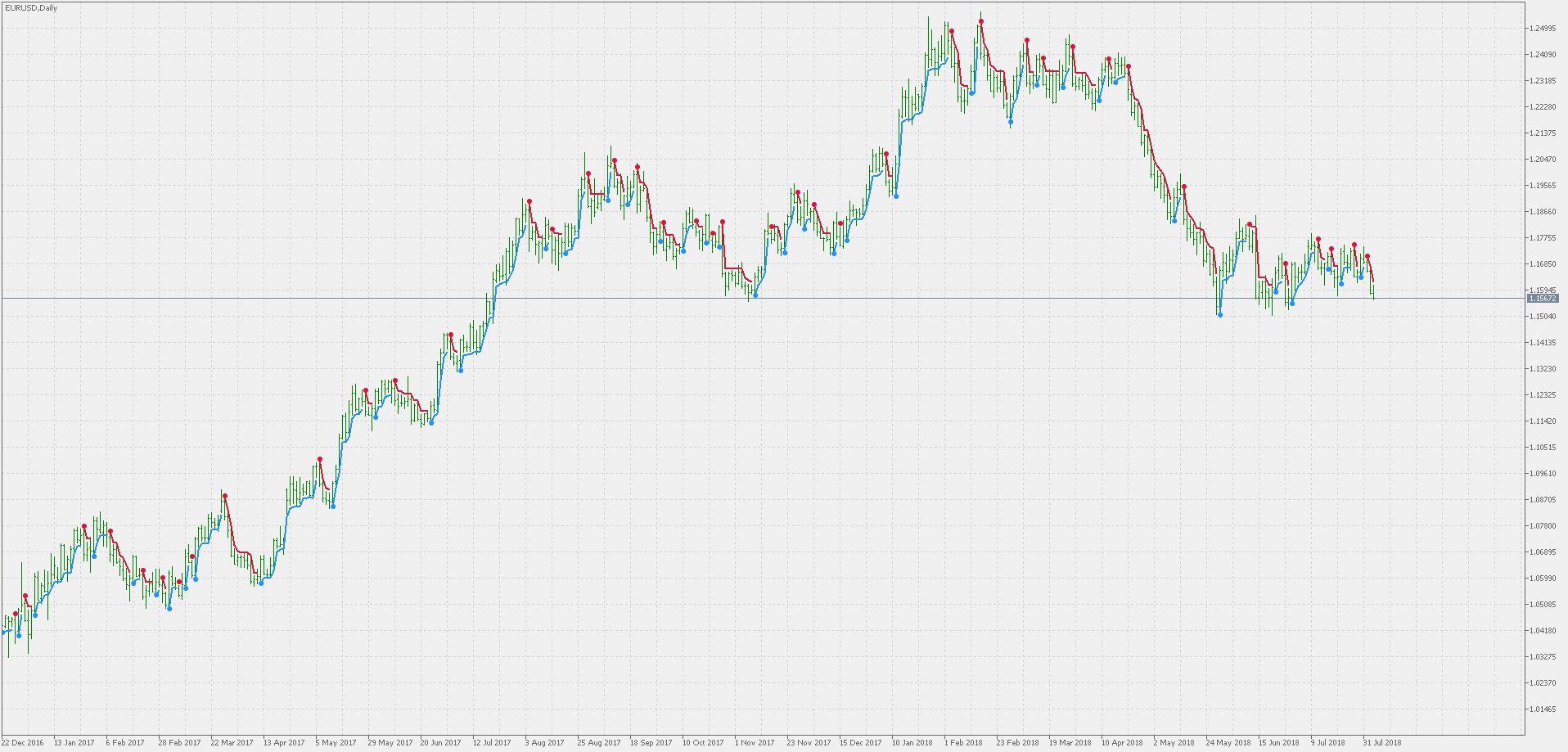Interesting script?
So post a link to it -
let others appraise it

You liked the script? Try it in the MetaTrader 5 terminal# ATR Trend Envelopes - indicator for MetaTrader 5

Views:
2750
Rating:
Published:
2018.08.06 17:16
Updated:
2018.12.01 20:01

Trend Envelopes indicator is calculated based on a % of price change. That % is then used to determine if there is a new trend or trend remains the same.

This version is using ATR for the price change calculation instead.The reason why the ATR is used is the following - the % change works OK for middle term timeframes. But for short timeframes and long timeframes it tends to be either too slow or too fast. Here is a comparison of this indicator (upper) and the "classical" version (lower) on daily timeframe. It is obvious that the difference is big and that the "trend" should be redefined in the "classical" calculation.QQE of Parabolic Weighted Velocity

This version of QQE is using Parabolic Weighted Velocity to determine trend.QQE of Velocity

This version of QQE is using Velocity indicator to determine trend.STD Trend Envelopes

Trend Envelopes indicator that uses Standard Deviation for the price change calculation.STD Trend Envelopes - RSI

This version of Trend Envelopes RSI indicator differs in one significant point: instead of using fixed value (5) for envelopes calculation, standard deviation (adjusted by multiplier) of the calculated RSI is used for envelopes calculation.